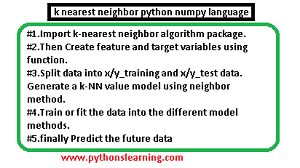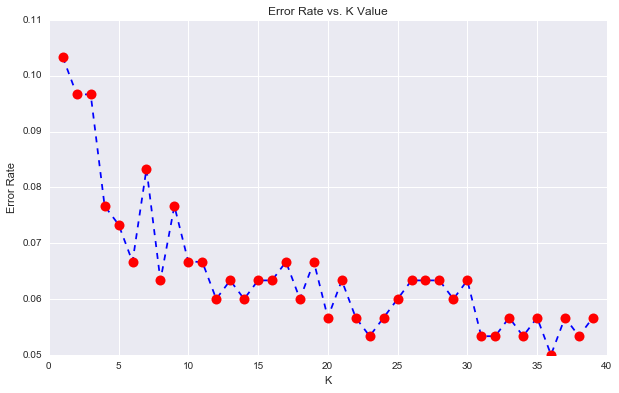# k nearest neighbor python numpy language:

Welcome everyone in python crash course (Machine learning). This is first part of this section,if you want to learn SVM in python then click on it.
K Nearest Neighbors method also used for data prediction purpose, so in his section we will learn  K Nearest Neighbors predict method.k nearest neighbor python numpy language

## How do you use K nearest neighbor in Python language in smart way?

In this project following steps are used to performed operation:
• Import knearest neighbor algorithm package.
• Then Create feature and target variables using function.
• Split data into x/y_training and x/y_test data.
• Generate a kNN value model using neighbor method.
• Train or fit the data into the different model methods.
• finally Predict the future data

### What is K nearest neighbor used for?

suppose you have been given a classified data set from a any popular company,they give you the data and the target classes and say predicts a class for a new data point based off of the features.
Let’s do it!

#### k nearest neighbor python 3.10

Import Libraries
import pandas as pd
import seaborn as sns
import matplotlib.pyplot as plt
import numpy as np
%matplotlib inline

Get the Data
Set index_col=0

WTS PTS EQS SBS LQS QWS FDS PJS HQS NXS TARGETC CLASSES
0.923917 1.172073 0.467946 0.655464 0.880862 0.252608 0.659697 0.343798 0.979422 1.231409 1
0.645632 1.033722 0.545342 0.865645 0.934109 0.658450 0.675334 1.213546 0.681552 1.492702 1
0.5521360 1.201493 0.921990 0.8775595 1.526629 0.720781 1.776351 1.154483 0.957877 1.285597 0
1.434204 1.386726 0.653046 0.425624 1.142504 0.875128 1.509708 1.380003 1.522692 1.253093 0
1.579491 0.949750 0.627280 0.768976 1.232537 0.703727 1.815596 0.646691 1.463812 1.519167 1

Standardize the Variables  K Nearest Neighbors method
In the next step we drop the TARGETC CLASSES from the data because this type of data affect on the prediction.

from sklearn.preprocessing import StandardScaler
scaler = StandardScaler()
scaler.fit(df.drop(‘TARGETC CLASSES’,axis=1))
StandardScaler(copy=True, with_mean=True, with_std=True)
scaled_features = scaler.transform(df.drop(‘TARGETC CLASSES’,axis=1))
df_feat = pd.DataFrame(scaled_features,columns=df.columns[:-1])

WTS  PTS EQS SBS LQS QWS FDS PJS HQS NXS
-0.045232 0.185907 -0.913431 0.3229629 -1.033637 -2.308375 -0.798951 -1.482368 -0.949719 -0.643314
-1.674836 -0.430348 -1.025313 0.625388 -0.444847 -1.152706 -1.129797 -0.202240 -1.828051 0.636759
-0.9988702 0.339318 0.301511 0.785873 2.031693 -0.870156 2.5109818 0.285707 -0.682494 -0.377850
0.992841 1.060193 -0.621399 0.635299 0.452820 -0.267220 1.770208 1.066491 1.241325 -1.026987
1.149275 -0.640392 -0.709819 -0.557175 0.822886 -0.936773 0.6996782 -1.472352 1.040772

Train Test Split method:

from sklearn.model_selection import train_test_split
X_train, X_test, y_train, y_test = train_test_split(scaled_features,df[‘TARGETC CLASSES’],
test_size=0.20)

Using KNN method

from sklearn.neighbors import KNeighborsClassifier
knn = KNeighborsClassifier(n_neighbors=1)
knn.fit(X_train,y_train)
KNeighborsClassifier(algorithm=’auto’, leaf_size=20, metric=’minkowski’,
metric_params=None, n_jobs=1, n_neighbors=1, p=2,
weights=’uniform’)
pred = knn.predict(X_test)

Predictions and Evaluations using knn
Let’s start to evaluate our KNN model!

from sklearn.metrics import classification_report,confusion_matrix

print(confusions_matrix(y_test,pred))

[[124  19]
[ 12 145]]

print(classification_report(y_test,pred))

precisionx    recallx  f1-scorex   supportx
1       0.92      0.87      0.89       144
0       0.99      0.92      0.90       158
avg / total       0.94      0.90      0.89       300

Choosing a correct value for K for this
we use the elbow method to pick a correct K Value:

error_rate = []

# wait some time

for i in range(1,40):
knn = KNeighborsClassifier(n_neighbors=i)
knn.fit(X_train,y_train)
pred_i = knn.predict(X_test)
error_rate.append(np.mean(pred_i != y_test))
plt.figure(figsize=(10,6))
plt.plot(range(1,40),error_rate,color=’blue’, linestyle=’dashed’, marker=’o’,
markerfacecolor=’red’, markersize=10)
plt.title(‘Error Rate value vs. K mean Value’)
plt.xlabel(‘K mean Value’)
plt.ylabel(‘Error Rate value’)<matplotlib.text.Text at 0x11ca93ba9>.
After run this program the knn plot is display
Summary:
In this section we saw the How to k nearest neighbor python numpy methods used in pyhon, so abou his secion any query then please comment.

see comp. between pyhon vs R vs…
Tags: k nearest neighbor python numpy,How do you use K nearest neighbor in Python language in smart way?,What is K nearest neighbor used for?,What is the Python code for importing k nearest Neighbours?
BEST OF LUCK!!

### sachin PagarI am Mr. Sachin pagar Embedded system engineer, the founder of Pythonslearning, a Passionate Educational Blogger and Author, who love to share the informative content on educational resources.

### sachin Pagar

I am Mr. Sachin pagar Embedded system engineer, the founder of Pythonslearning, a Passionate Educational Blogger and Author, who love to share the informative content on educational resources.